Publicité

# Ijcse13 05-01-048

lecturer at gayatri college à gayatri college
16 Nov 2013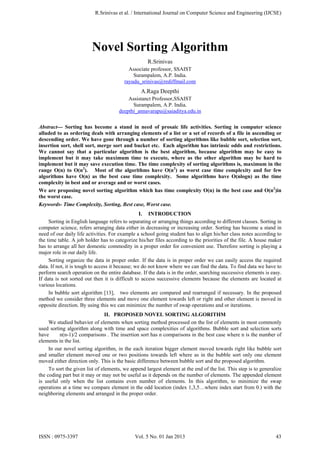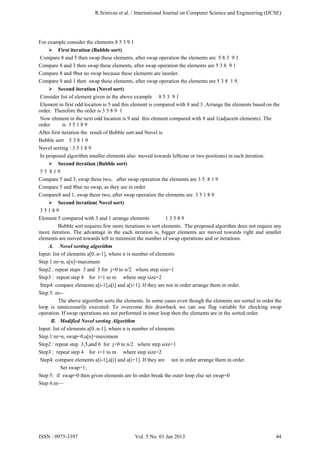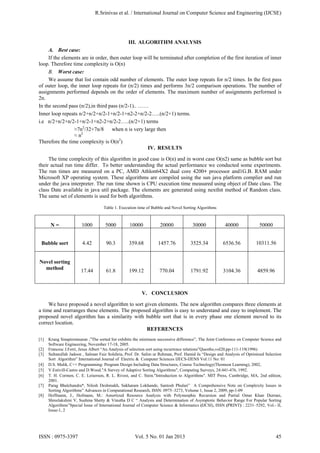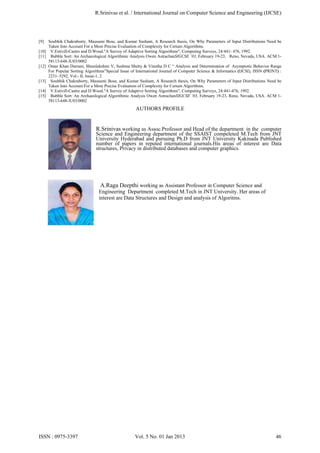Prochain SlideShare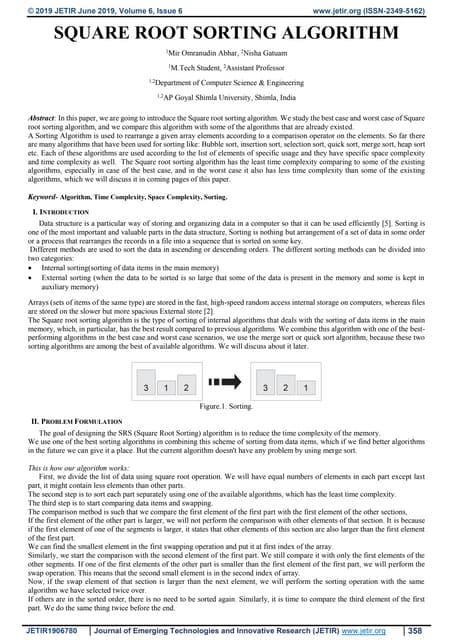SQUARE ROOT SORTING ALGORITHM
Chargement dans ... 3
1 sur 4
Publicité

### Ijcse13 05-01-048

1. R.Srinivas et al. / International Journal on Computer Science and Engineering (IJCSE) Novel Sorting Algorithm R.Srinivas Associate professor, SSAIST Surampalem, A.P. India. rayudu_srinivas@rediffmail.com A.Raga Deepthi Assistanct Professor,SSAIST Surampalem, A.P. India. deepthi_annavarapu@saiaditya.edu.in Abstract--- Sorting has become a stand in need of prosaic life activities. Sorting in computer science alluded to as ordering deals with arranging elements of a list or a set of records of a file in ascending or descending order. We have gone through a number of sorting algorithms like bubble sort, selection sort, insertion sort, shell sort, merge sort and bucket etc. Each algorithm has intrinsic odds and restrictions. We cannot say that a particular algorithm is the best algorithm, because algorithm may be easy to implement but it may take maximum time to execute, where as the other algorithm may be hard to implement but it may save execution time. The time complexity of sorting algorithms is, maximum in the range O(n) to O(n2). Most of the algorithms have O(n2) as worst case time complexity and for few algorithms have O(n) as the best case time complexity. Some algorithms have O(nlogn) as the time complexity in best and or average and or worst cases. We are proposing novel sorting algorithm which has time complexity O(n) in the best case and O(n2)in the worst case. Keywords- Time Complexity, Sorting, Best case, Worst case. I. INTRODUCTION Sorting in English language refers to separating or arranging things according to different classes. Sorting in computer science, refers arranging data either in decreasing or increasing order. Sorting has become a stand in need of our daily life activities. For example a school going student has to align his/her class notes according to the time table. A job holder has to categorize his/her files according to the priorities of the file. A house maker has to arrange all her domestic commodity in a proper order for convenient use. Therefore sorting is playing a major role in our daily life. Sorting organize the data in proper order. If the data is in proper order we can easily access the required data. If not, it is tough to access it because; we do not know where we can find the data. To find data we have to perform search operation on the entire database. If the data is in the order, searching successive elements is easy. If data is not sorted out then it is difficult to access successive elements because the elements are located at various locations. In bubble sort algorithm , two elements are compared and rearranged if necessary. In the proposed method we consider three elements and move one element towards left or right and other element is moved in opposite direction. By using this we can minimize the number of swap operations and or iterations. II. PROPOSED NOVEL SORTING ALGORITHM We studied behavior of elements when sorting method processed on the list of elements in most commonly used sorting algorithm along with time and space complexities of algorithms. Bubble sort and selection sorts have n(n-1)/2 comparisons . The insertion sort has n comparisons in the best case where n is the number of elements in the list. In our novel sorting algorithm, in the each iteration bigger element moved towards right like bubble sort and smaller element moved one or two positions towards left where as in the bubble sort only one element moved either direction only. This is the basic difference between bubble sort and the proposed algorithm. To sort the given list of elements, we append largest element at the end of the list. This step is to generalize the coding part but it may or may not be useful as it depends on the number of elements. The appended element is useful only when the list contains even number of elements. In this algorithm, to minimize the swap operations at a time we compare element in the odd location (index 1,3,5…where index start from 0.) with the neighboring elements and arranged in the proper order. ISSN : 0975-3397 Vol. 5 No. 01 Jan 2013 43
2. R.Srinivas et al. / International Journal on Computer Science and Engineering (IJCSE) For example consider the elements 8 5 3 9 1  First iteration (Bubble sort) Compare 8 and 5 then swap these elements, after swap operation the elements are 5 8 3 9 1 Compare 8 and 3 then swap these elements, after swap operation the elements are 5 3 8 9 1 Compare 8 and 9but no swap because these elements are inorder. Compare 9 and 1 then swap these elements, after swap operation the elements are 5 3 8 1 9.  Second iteration (Novel sort) Consider list of element given in the above example 8 5 3 9 1 Element in first odd location is 5 and this element is compared with 8 and 3 .Arrange the elements based on the order. Therefore the order is 3 5 8 9 1 Now element in the next odd location is 9 and this element compared with 8 and 1(adjacent elements). The order is 3 5 1 8 9 After first iteration the result of Bubble sort and Novel is Bubble sort 5 3 8 1 9 Novel sorting : 3 5 1 8 9 In proposed algorithm smaller elements also moved towards left(one or two positions) in each iteration.  Second iteration (Bubble sort) 53 819 Compare 5 and 3, swap these two, after swap operation the elements are 3 5 8 1 9 Compare 5 and 8but no swap, as they are in order Compare8 and 1, swap these two, after swap operation the elements are 3 5 1 8 9  Second iteration( Novel sort) 35189 Element 5 compared with 3 and 1 arrange elements 13589 Bubble sort requires few more iterations to sort elements. The proposed algorithm does not require any more iteration. The advantage in the each iteration is, bigger elements are moved towards right and smaller elements are moved towards left to minimize the number of swap operations and or iterations. A. Novel sorting algorithm Input: list of elements a[0..n-1], where n is number of elements Step 1:m=n, a[n]=maximum Step2 : repeat steps 3 and 5 for j=0 to n/2 where step size=1 Step3 : repeat step 4 for i=1 to m where step size=2 Step4: compare elements a[i-1],a[i] and a[i+1]. If they are not in order arrange them in order. Step 5: m-The above algorithm sorts the elements. In some cases even though the elements are sorted in order the loop is unnecessarily executed. To overcome this drawback we can use flag variable for checking swap operation. If swap operations are not performed in inner loop then the elements are in the sorted order. B. Modified Novel sorting Algorithm Input: list of elements a[0..n-1], where n is number of elements Step 1:m=n, swap=0,a[n]=maximum Step2 : repeat step 3,5,and 6 for j=0 to n/2 where step size=1 Step3 : repeat step 4 for i=1 to m where step size=2 Step4: compare elements a[i-1],a[i] and a[i+1]. If they are not in order arrange them in order. Set swap=1; Step 5: if swap=0 then given elements are In order break the outer loop else set swap=0 Step 6:m— ISSN : 0975-3397 Vol. 5 No. 01 Jan 2013 44
3. R.Srinivas et al. / International Journal on Computer Science and Engineering (IJCSE) III. ALGORITHM ANALYSIS A. Best case: If the elements are in order, then outer loop will be terminated after completion of the first iteration of inner loop. Therefore time complexity is O(n) B. Worst case: We assume that list contain odd number of elements. The outer loop repeats for n/2 times. In the first pass of outer loop, the inner loop repeats for (n/2) times and performs 3n/2 comparison operations. The number of assignments performed depends on the order of elements. The maximum number of assignments performed is 2n. In the second pass (n/2),in third pass (n/2-1).. …… Inner loop repeats n/2+n/2+n/2-1+n/2-1+n2-2+n/2-2…..(n/2+1) terms. i.e n/2+n/2+n/2-1+n/2-1+n2-2+n/2-2…..(n/2+1) terms ≈7n2/32+7n/8 when n is very large then ≈ n2 Therefore the time complexity is O(n2) IV. RESULTS The time complexity of this algorithm in good case is O(n) and in worst case O(n2) same as bubble sort but their actual run time differ. To better understanding the actual performance we conducted some experiments. The run times are measured on a PC, AMD Athlon64X2 dual core 4200+ processor and1G.B. RAM under Microsoft XP operating system. These algorithms are compiled using the sun java platform complier and run under the java interpreter. The run time shown is CPU execution time measured using object of Date class. The class Date available in java util package. The elements are generated using nextInt method of Random class. The same set of elements is used for both algorithms. Table 1. Execution time of Bubble and Novel Sorting Algorithms N= 1000 5000 10000 20000 30000 40000 50000 Bubble sort 4.42 90.3 359.68 1457.76 3525.34 6536.56 10311.56 Novel sorting method 17.44 61.8 199.12 770.04 1791.92 3104.36 4859.96 V. CONCLUSION We have proposed a novel algorithm to sort given elements. The new algorithm compares three elements at a time and rearranges these elements. The proposed algorithm is easy to understand and easy to implement. The proposed novel algorithm has a similarity with bubble sort that is in every phase one element moved to its correct location. REFERENCES         Krung Sinapiromsaran ,”The sorted list exhibits the minimum successive difference”, The Joint Conference on Computer Science and Software Engineering, November 17-18, 2005. Francesc J.Ferri, Jesus Albert “An Analysis of selection sort using recurrence relations”Questho,vol20,pp-111-119(1996) Sultanullah Jadoon , Salman Faiz Solehria, Prof. Dr. Salim ur Rehman, Prof. Hamid Ja “Design and Analysis of Optimized Selection Sort Algorithm” International Journal of Electric & Computer Sciences IJECS-IJENS Vol:11 No: 01 D.S. Malik, C++ Programming: Program Design Including Data Structures, Course Technology(Thomson Learning), 2002, V.Estivill-Castro and D.Wood."A Survey of Adaptive Sorting Algorithms", Computing Surveys, 24:441-476, 1992. T. H. Cormen, C. E. Leiserson, R. L. Rivest, and C. Stein."Introduction to Algorithms". MIT Press, Cambridge, MA, 2nd edition, 2001. Parag Bhalchandra*, Nilesh Deshmukh, Sakharam Lokhande, Santosh Phulari” A Comprehensive Note on Complexity Issues in Sorting Algorithms” Advances in Computational Research, ISSN: 0975–3273, Volume 1, Issue 2, 2009, pp-1-09 Hoffmann, J., Hofmann, M.: Amortized Resource Analysis with Polymorphic Recursion and Partial Omar Khan Durrani, Shreelakshmi V, Sushma Shetty & Vinutha D C “ Analysis and Determination of Asymptotic Behavior Range For Popular Sorting Algorithms”Special Issue of International Journal of Computer Science & Informatics (IJCSI), ISSN (PRINT) : 2231–5292, Vol.- II, Issue-1, 2 ISSN : 0975-3397 Vol. 5 No. 01 Jan 2013 45
4. R.Srinivas et al. / International Journal on Computer Science and Engineering (IJCSE)        Soubhik Chakraborty, Mausumi Bose, and Kumar Sushant, A Research thesis, On Why Parameters of Input Distributions Need be Taken Into Account For a More Precise Evaluation of Complexity for Certain Algorithms. V.Estivill-Castro and D.Wood."A Survey of Adaptive Sorting Algorithms", Computing Surveys, 24:441- 476, 1992. Bubble Sort: An Archaeological Algorithmic Analysis Owen AstrachanSIGCSE ’03, February 19-23, Reno, Nevada, USA. ACM 158113-648-X/03/0002 Omar Khan Durrani, Shreelakshmi V, Sushma Shetty & Vinutha D C “ Analysis and Determination of Asymptotic Behavior Range For Popular Sorting Algorithms”Special Issue of International Journal of Computer Science & Informatics (IJCSI), ISSN (PRINT) : 2231–5292, Vol.- II, Issue-1, 2 Soubhik Chakraborty, Mausumi Bose, and Kumar Sushant, A Research thesis, On Why Parameters of Input Distributions Need be Taken Into Account For a More Precise Evaluation of Complexity for Certain Algorithms. V.Estivill-Castro and D.Wood."A Survey of Adaptive Sorting Algorithms", Computing Surveys, 24:441-476, 1992. Bubble Sort: An Archaeological Algorithmic Analysis Owen AstrachanSIGCSE ’03, February 19-23, Reno, Nevada, USA. ACM 158113-648-X/03/0002 AUTHORS PROFILE R.Srinivas working as Assoc.Professor and Head of the department in the computer Science and Engineering department of the SSAIST compeleted M.Tech from JNT University Hyderabad and pursuing Ph.D from JNT University Kakinada Published number of papers in reputed international journals.His areas of interest are Data structures, Privacy in distributed databases and computer graphics. A.Raga Deepthi working as Assistant Professor in Computer Science and Engineering Department completed M.Tech in JNT University. Her areas of interest are Data Structures and Design and analysis of Algoritms. ISSN : 0975-3397 Vol. 5 No. 01 Jan 2013 46
Publicité